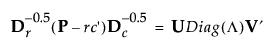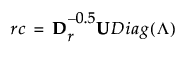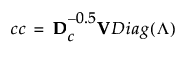Publication date: 11/10/2021

## Details Report in Correspondence Analysis

Lists values from the following singular value decomposition (SVD):where:

P is the matrix of counts divided by the total frequency

r and c are row and column sums of P

the D matrices are diagonal matrices of the values of r and c

Λ is the column vector of the singular values reported in the details report